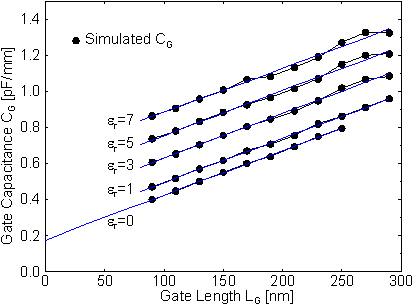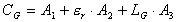Next: 6.1.2.3 Reduction of the Recess Length Up: 6.1.2 RF Characteristics Previous: 6.1.2.1 Reduction of the Gate Length

6.1.2.2 Contributions to the Gate Capacitance

From the dependence on the gate length the different contributions to the total gate capacitance can be separated by simulation. We change the capacitive coupling of the gate metal to the ohmic contacts and to the semiconductor material by setting the permittivity of the passivation to different values. er = 1 corresponds to no passivation at all. The opposite extreme is represented by the case er = 7 (space between contacts completely filled with dielectrics).  Figure 6.16 shows the simulated CG as a function of LG for er = 0, 1 3 5 and 7. A linear fit can be found for all simulated CG versus LG.Figure 6.16 Simulated gate capacitance CG vs. gate length LG for two different er at VDS = 2.0 V, ID = 160 mA/mm.

The separation of the lines also reveals a linear dependence on er. Thus, CG can be described by the expression. (62)

By extrapolation of the straight line for er = 1 to LG = 0, the fringe capacitance CF = A1 + A2 » 243 fF/mm is obtained. Because the two-dimensional gate cross section is fully taken into account, this value is higher than the one given by Wasserstrom and McKenna  who use infinitely thin contacts in their model. In the simulation er can be even decreased to zero. The linear interpolation of CG for er = 0 gives A1 = 177 fF/mm. A1 corresponds to the fringe capacitance purely attributed to the semiconductor which depends only on the epitaxial structure and the separations of source, gate, and drain contacts.

From the separations of the straight lines in  Figure 6.16, A2 can be deduced. This contribution is determined by the device cross section above the semiconductor surface. In our case, A2 » 66 fF/mm. Finally, A3 is given by the slope of the lines. Apart from some influences of minor importance (like lateral spacing of the three contacts), this quantity is dominated by the sheet charge under the gate. In our example, A3 = 2.4 nF/mm2 (for a gate with LG = 100 nm, this is equivalent to 240 fF/mm). This last term in (62) is the only one necessary for the function of the transistor, the other two only reduce its performance.Next: 6.1.2.3 Reduction of the Recess Length Up: 6.1.2 RF Characteristics Previous: 6.1.2.1 Reduction of the Gate Length

Helmut Brech
1998-03-11